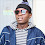# How to drop one or multiple columns from Pandas Dataframe

In this tutorial, we will cover how to drop or remove one or multiple columns from pandas dataframe.
What is pandas in Python?
pandas is a python package for data manipulation. It has several functions for the following data tasks:
1. Drop or Keep rows and columns
2. Aggregate data by one or more columns
3. Sort or reorder data
4. Merge or append multiple dataframes
5. String Functions to handle text data
6. DateTime Functions to handle date or time format columns
To make use of any python library, we first need to load them up by using `import` command.
```import pandas as pd
import numpy as np
```
Let's create a fake dataframe for illustration
The code below creates 4 columns named A through D.
```df = pd.DataFrame(np.random.randn(6, 4), columns=list('ABCD'))
```
```          A         B         C         D
0 -1.236438 -1.656038  1.655995 -1.413243
1  0.507747  0.710933 -1.335381  0.832619
2  0.280036 -0.411327  0.098119  0.768447
3  0.858730 -0.093217  1.077528  0.196891
4 -0.905991  0.302687  0.125881 -0.665159
5 -2.012745 -0.692847 -1.463154 -0.707779
```

## Drop a column in python

In pandas, `drop( )` function is used to remove column(s).`axis=1` tells Python that you want to apply function on columns instead of rows.
`df.drop(['A'], axis=1)`
Column A has been removed. See the output shown below.
```          B         C         D
0 -1.656038  1.655995 -1.413243
1  0.710933 -1.335381  0.832619
2 -0.411327  0.098119  0.768447
3 -0.093217  1.077528  0.196891
4  0.302687  0.125881 -0.665159
5 -0.692847 -1.463154 -0.707779
```
In order to create a new dataframe `newdf` storing remaining columns, you can use the command below.
`newdf = df.drop(['A'], axis=1)`
To delete the column permanently from original dataframe `df`, you can use the option `inplace=True`
```df.drop(['A'], axis=1, inplace=True)
```
```#Check columns in df after dropping column A
df.columns

Output
Index(['B', 'C', 'D'], dtype='object')
```
The parameter `inplace=` can be deprecated (removed) in future which means you might not see it working in the upcoming release of pandas package. You should avoid using this parameter if you are not already habitual of using it. Instead you can store your data after removing columns in a new dataframe (as explained in the above section).

If you want to change the existing dataframe, try this ` df = df.drop(['A'], axis=1) `

## Remove Multiple Columns in Python

You can specify all the columns you want to remove in a list and pass it in `drop( )` function.
Method I
```df2 = df.drop(['B','C'], axis=1)
```
Method II
```cols = ['B','C']
df2 = df.drop(cols, axis=1)
```
Select or Keep Columns
If you wish to select a column (instead of drop), you can use the command
`df['A']`
To select multiple columns, you can submit the following code.
`df[['A','B']]`

## How to drop column by position number from pandas Dataframe?

You can find out name of first column by using this command `df.columns`. Indexing in python starts from 0.
```df.drop(df.columns, axis =1)
```
To drop multiple columns by position (first and third columns), you can specify the position in list `[0,2]`.
```cols = [0,2]
df.drop(df.columns[cols], axis =1)
```

## Drop columns by name pattern

`df = pd.DataFrame({"X1":range(1,6),"X_2":range(2,7),"YX":range(3,8),"Y_1":range(2,7),"Z":range(5,10)})`
```   X1  X_2  YX  Y_1  Z
0   1    2   3    2  5
1   2    3   4    3  6
2   3    4   5    4  7
3   4    5   6    5  8
4   5    6   7    6  9
```

### Drop column whose name starts with letter 'X'

```df.loc[:,~df.columns.str.contains('^X')]
```
How it works?
1. `^X` is a expression of regex language which refers to beginning of letter 'X'
2. `df.columns.str.contains('^X')` returns array [True, True, False, False, False].
True where condition meets. Otherwise False
3. Sign `~` refers to negate the condition.
4. `df.loc[ ]` is used to select columns
It can also be written like :
`df.drop(df.columns[df.columns.str.contains('^X')], axis=1)`
Other Examples
```#Removing columns whose name contains string 'X'
df.loc[:,~df.columns.str.contains('X')]

#Removing columns whose name contains string either 'X' or 'Y'
df.loc[:,~df.columns.str.contains('X|Y')]

#Removing columns whose name ends with string 'X'
df.loc[:,~df.columns.str.contains('X\$')]
```

### Drop columns where percentage of missing values is greater than 50%

```df = pd.DataFrame({'A':[1,3,np.nan,5,np.nan],
'B':[4,np.nan,np.nan,5,np.nan]
})
```
% of missing values can be calculated by mean of NAs in each column.
```cols = df.columns[df.isnull().mean()>0.5]
df.drop(cols, axis=1)
```
Related Posts
ShareDeepanshu founded ListenData with a simple objective - Make analytics easy to understand and follow. He has over 10 years of experience in data science. During his tenure, he worked with global clients in various domains like Banking, Insurance, Private Equity, Telecom and HR.

16 Responses to "How to drop one or multiple columns from Pandas Dataframe"
1.Careful of the API future of `inplace` https://github.com/pandas-dev/pandas/issues/16529

1.Thanks for highlighting the same. I added it in the post to discourage the use of it. Cheers!

2.1.3.Great...article simple and concise...

1.4.Thanks for a nice article. Now, a request. If you find time, can you also write on operations on rows.

5.Thanks for nice article..

6.Sure. Thank you for stopping by my blog!

7.can u tell me how to apply only one feature on your dataset with code.i hope u you will response as soon as possible.

8.Really easy to understand sir !!!

9.great tips, very well presented and easy to understand ! thanks !

10.great; this really helped me a lot as a beginner.

11.Nice one..great job

12.Hi I have removed missing values from dataset permanently by using inplace=True. i want to restore back to original data frame data,how do i do that

13.Thanks for helping out

Next → ← Prev
Looks like you are using an ad blocker!

To continue reading you need to turnoff adblocker and refresh the page. We rely on advertising to help fund our site. Please whitelist us if you enjoy our content.